# Algorithms, combinations of

(diff) ← Older revision | Latest revision (diff) | Newer revision → (diff)

The name of several methods for constructing new algorithms (cf. Algorithm) from given algorithms.

As applied to normal algorithms (cf. Normal algorithm), the following combinations (compositions) are best known: normal composition () of two normal algorithmsand, normal union (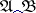) of two normal algorithmsand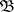, normal branching () of two normal algorithmsandcontrolled by a normal algorithm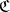, and normal repetition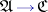of a normal algorithmcontrolled by a normal algorithm. Ifandare normal algorithms in some alphabet, the above combinations are normal algorithms in a certain given extension ofand satisfy the following conditions: a) for any word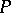in,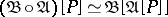is true (the composition theorem); b) for any wordin,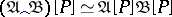is true (the union theorem); c) for any wordin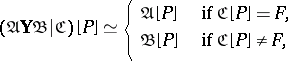moreover, ifhas been defined, then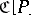is defined as well (the branching theorem); d) for any wordsandin, the graphic equality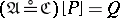is true if and only if it is possible to indicate a sequence of words() over the alphabetsuch that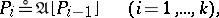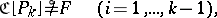(the repetition theorem). Similar theorems may also be obtained for Turing machines (cf. Turing machine). In the theory of recursive functions (cf. Recursive function), the combinations of these functions which are most frequently employed are those supplied by the substitution operator, the primitive recursion operator and the-operator.

Theorems on combinations of algorithms reveal an important feature of the existing formalizations of the general concept of an algorithm — to wit, their "closure" under the natural ways of combination of algorithms. This fact is one of the principal arguments in favour of the basic assumption about algorithms (the Church thesis). Theorems on compositions of algorithms are an important part of the general theory of algorithms. Having been demonstrated once, they make it possible to determine the realizability of complicated and cumbersome algorithms, without actually writing down their schemes.

Of major interest in the general theory of algorithms is the problem of synthesizing an arbitrary algorithm within some class of interest, using a pre-determined set of composition operators.

How to Cite This Entry:
Algorithms, combinations of. Encyclopedia of Mathematics. URL: http://encyclopediaofmath.org/index.php?title=Algorithms,_combinations_of&oldid=16682
This article was adapted from an original article by N.M. Nagornyi (originator), which appeared in Encyclopedia of Mathematics - ISBN 1402006098. See original article